# Exploratory factor analysis

### Introduction

Exploratory factor analysis is utilised to identify sets of correlated items, which might ultimately be applied to construct scales. This technique uncovers latent variables called factors.

To illustrate, consider a researcher who wants to ascertain whether or not individuals with various hairstyles are more likely to engage in peculiar behaviours. Specifically, the researcher seeks individuals with mullets, comb-overs, perms, and mohawks. Individuals are asked to estimate the extent to which they undertake nine peculiar activities, on scale that ranges from 1 to 5. In particular, they are asked to estimate the extent to which they listen to Rolf Harris, discuss brands of nail clippers, enjoy statistics, store ear wax, examine gunk under toe nails, display their double jointed arms, ring people at 3.00 am, go home when it is their turn to shout drinks, and play music at maximum volume. An extract of the data is presented below.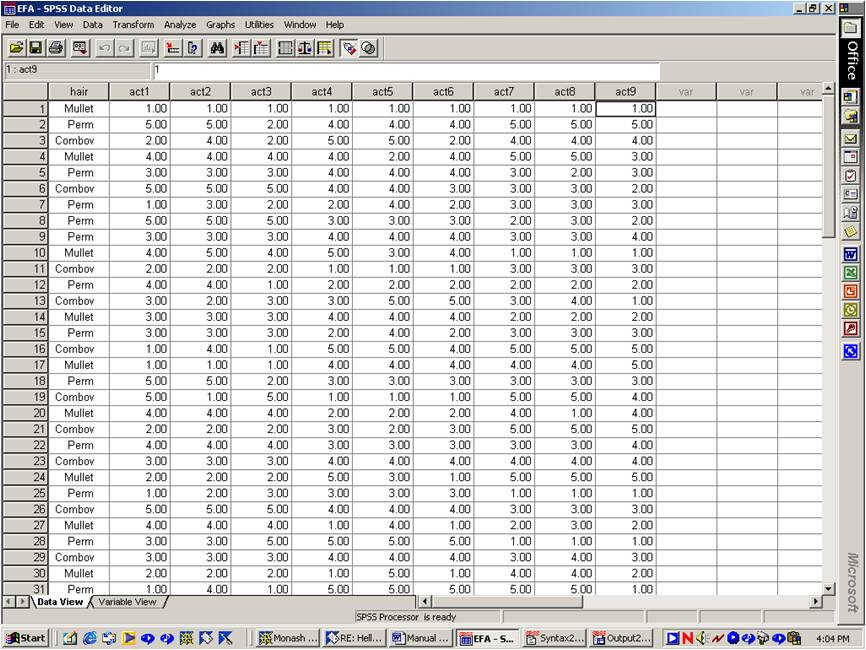To determine whether or not hairstyle influences behaviour, a series of ANOVAs could be undertaken. Each ANOVA could pertain to a separate act or behaviour. The pattern of results that might emerge from these ANOVAs is likely to be complex, unclear, and thus difficult to interpret.

Alternatively, a MANOVA or discriminant function analysis could be undertaken to clarify interpretations. Unfortunately, this analysis is probably unsuitable here. In particular, some of the behaviours are likely to be highly correlated. For example, individuals who ring other individuals late at night also tend to play music at a high volume. The pronounced correlation between these acts creates a problem called multicolinearity, which tends to undermine the power of MANOVA and discriminant function analysis.

Clearly, to resolve these difficulties, the 9 behaviors somehow need to be integrated into a more manageable set of variables. In particular:

• The researcher needs to identify sets or clusters of behaviours that tend to coincide or correlate with one another.
• Behaviors in each set can then be averaged or combined together.
• To identify clusters of behaviors that tend to correlated with one another, the researcher could simply explore the correlations between each pair of acts.
• This matrix of correlations is presented below.In this example, Acts 1, 2 and 3 seem to be highly correlated with one another. These items reflect the extent to which individuals listen to Rolf Harris, discuss brands of nail clippers, and enjoy statistics. Hence, these items perhaps reflect the extent to which the individual is boring.

Likewise, Acts 4, 5 and 6 seem to be highly correlated with one another. These items reflect the extent to which individuals store ear wax, examine gunk under toe nails, and display their double jointed arms. Accordingly, these items perhaps reflect the extent to which the individual is vulgar.

Finally, Acts 7, 8 and 9 also to be highly correlated with one another. These items reflect the extent to which individuals ring people at 3.00 am, go home when it is their turn to shout drinks, and play music at maximum volume. In other words, these items perhaps reflect the extent to which the individual is insensitive.

In other words, researchers could potentially average the first three items to generate a measure of boring behaviour. The same process could be applied to the other clusters of items. All the analyses can then be restricted to these three new columns of scores, which are presented below.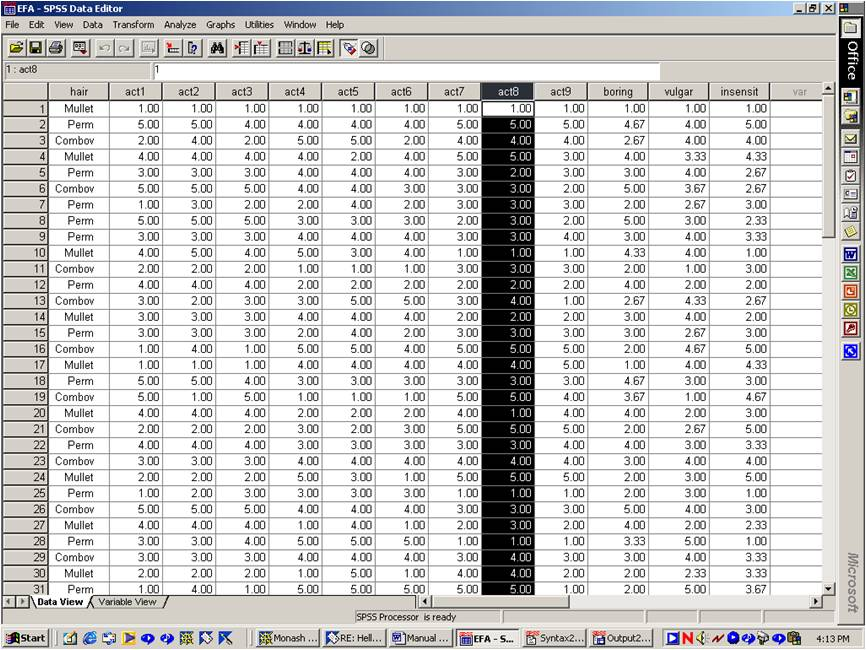Unfortunately, the results are seldom as unambiguous and simple as these correlations. Hence, a formal, systematic procedure is needed to explore this matrix of correlations to identify factors or clusters of items that correlate with one another. Exploratory factor analysis provides this function. In a nutshell, exploratory factor analysis is utilised to uncover sets of items that correlate with one another and thus reflect a similar construct or trait.

### Step 1: Administer the factor analysis

To undertake an exploratory factor analysis:

1. Select "Dimension reduction" and then "Factor" from the "Analyse Data" menu, after opening the appropriate data file in SPSS
2. Select the variables from which you would like to extract factors into the box labelled "Variables"
3. Press the button labelled "Extraction".
4. Stipulate the extraction method. Many researchers select "Principal Axis Factoring" if they would like to utilise the insights that emerge from this analysis in future studies& otherwise, they often select "Principal Components Analysis". The various methods will be broached later. Press "Continue".
5. Press the button labelled "Rotation".
6. Stipulate the rotation method. Many researchers utilise the "varimax" option. The various alternatives will be differentiated later.
7. Press OK.

### Step 2: Determine the ideal number of factors

The first step is to ascertain the number of factors or clusters to which the items belong. For example, perhaps the data comprise two factors. That is, items 1 to 5 might correlate with one another and thus represent one factor. In addition, items 6 to 9 might correlate with one another and thus represent another factor. Alternatively, the data might comprise thee, four, or even five factors.

In reality, the ideal number of factors cannot be determined definitively. Nevertheless, SPSS provides some information that can be utilized to estimate the number of factors that should be retained. This information is presented in a table called total variance explained, and is presented below.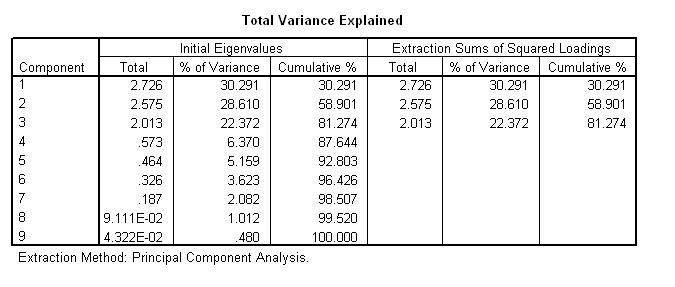To ascertain the ideal number of factors, consult the first set of three columns labelled "Initial eigenvalues". The subcolumn labelled "Total" presents the eigenvalues. These eigenvalues and the adjacent percentages of variance reflect the importance associated with each of the 9 factors. In essence, these indices reflect the extent to which each factors explains variance in the original responses. To illustrate this concept, suppose the researcher only utilised individuals who were equivalent on some factor. For example, the researcher might only utilise individuals who are equally vulgar.

The following table presents the responses associated with this sample of individuals. These participants are somewhat homogenous and hence their responses are clearly less variable than responses provided in the original sample. The variance may decline from 3.0 to 2.0, for example. Accordingly, the factor associated with vulgarity explains 30% of the variance in the original scores.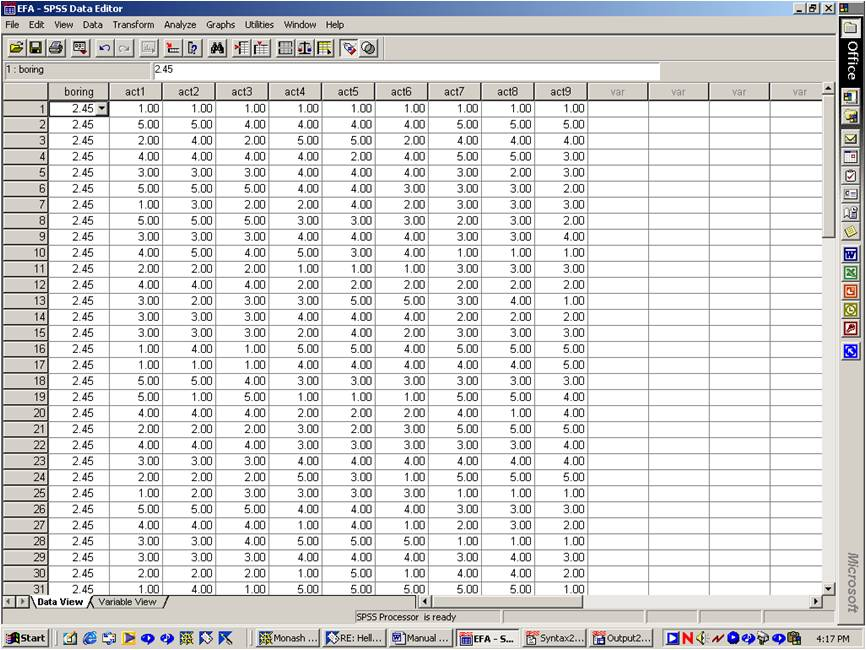Researchers always discard factors in which the eigenvalue is less than one. By definition, these factors explain less variance than a single item. Using the previous set of eigenvalues, researchers would thus discard Factors 4 to 9 and thus retain only 3 factors. Nevertheless, researchers do not always retain all the factors in which the corresponding eigenvalue exceeds one. In particular:

• For example, suppose factors 6, 7 and 8 yield eigenvalues that exceed one but are virtually identical.
• The similarity of these factors implies the eigenvalues have reached an asymptote or minimum plateau.
• Hence, these factors could perhaps be discarded.
• Alternatively, some researchers exclude factors in which the corresponding percentage of variance explained is less than 5%.
• In addition, some researchers create the scree plot--one of the options. All the factors that correspond to the same plateau are discarded.
• Finally, some researchers examine the residual correlation matrix, a concept that will be addressed later.

### Optional Step 3: Execute factor analysis again

The previous section revealed some procedures that are followed to identify the ideal number of factors. By default, SPSS rotates and thus explores all the factors in which the eigenvalue exceeded one. However, if the researcher decides the ideal number of factors should be less than the number of eigenvalues that exceed one, an additional analysis needs to be completed. Specifically, the researcher must repeat the factor analysis, after specifying the number of factors that should be retained, using the following process:

1. Undertake the factor analysis again, using the process that was outlined earlier
2. Before executing the OK button, press the button labelled "Extract"
3. Tick the option "Number of factors". Specify the ideal number of factors to explore.

### Step 4: Examine the factor matrix

Once the number of factors has been determined, and the analysis has been repeated if necessary, the researcher should examine the table entitled "Factor matrix". This table, which is presented below, indicates the extent to which each item pertains to a particular factor or cluster of variables. Specifically, values above approximately 0.32--or below approximately -0.32--indicate the item is strongly correlated with the corresponding factor. For example, in this instance, Act 2 seems to pertain to Factor 1 and not Factor 2 or 3. When the sample size is less than 200, researchers often increase the criterion to 0.44 or even higher.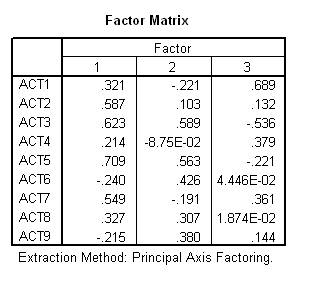Unfortunately, the factor matrix seldom presents useful outcomes. For example, in this instance, Act 3 seems to pertain to all the factors. Act 9 seems to pertain to none of the factors, and so forth. In other words, these findings do not yield three distinct clusters of items, as desired. Accordingly:

1. Researchers tend to disregard the factor matrix.
2. However, sometimes all the items seem to correspond to the first factor only.
3. This finding indicates that perhaps only one factor underlies the data.
4. Otherwise, the factor matrix does not provide informative data.

### Step 5: Examine rotated matrix

SPSS thus undertakes a procedure called rotation to circumvent this limitation. The objective of rotation is straightforward. Essentially, this procedure is designed to ensure that all the numbers in the matrix, which are usually called factor loadings, are either very low or very high to expedite interpretations. Specifically, this procedure attempts to shift the factor loadings closer to -1, 0, or 1. For example, a typical rotated matrix is presented below. In this instance:

1. Acts 1, 2, and 3 clearly pertain to one factor.
2. Hence, these items correspond to one set of correlated variables.
3. Likewise, Acts 4, 5 and 6 clearly pertain to another factor and thus represent a second set of correlated variables.
4. Finally, Acts 7, 8, and 9 pertain to a third factor.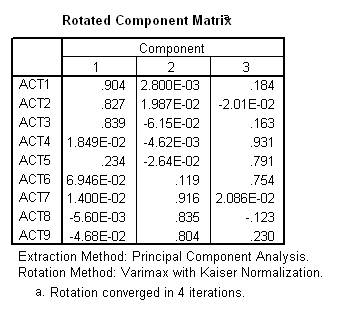### Step 6: Create scores and examine reliability

To reiterate, the previous analyses generated three factors. Most researchers undertake additional analyses to verify the items within each factor are indeed correlated sufficiently. Specifically, they examine the internal consistency of each factor in sequence, using Cronbach's alpha. To implement this procedure:

1. Select "Scale" and then "Reliability analysis" from the "Analyse" menu
2. Transfer the items associated with one scale, such as Acts 1, 2, and 3, to the box labelled "Variables"
3. Press "Statistics" and tick "Scale if item deleted". This option presents the Cronbach's alpha that would emerge after each individual item is deleted.
4. Press Continue and OK.
5. Apply the same process to each set of items in turn.

Ideally, each Cronbach's alpha should exceed 0.7. If not, some items or even factors can be rejected to raise power. If Cronbach's alpha is especially low, you might have overlooked the possibility that some, but not all, the items might be inversely related to the factor. For instance, suppose one of the acts was "refrain from playing loud music". In this instance, higher scores on this item reflect lower levels of insensitive behaviour. Accordingly, these variables must be recoded or modified. To modify these items appropriately:

1. Select "Compute" from the "Transform" menu.
2. For this item, type a label, such as "Act9r", in the box called "Target variable"
3. Type an equation, such as "6 - Act9", in the box called "Numeric expression". That is, subtract the scores on this item (e.g. Act9) from a number that exceeds the highest possible rating by 1 (e.g. 6).
4. Press OK.

This process reverses the scale and ensures that higher numbers, in this example, represent more pronounced levels of insensitive behaviour. Once the researcher has decided which items to retain, they usually average or sum the variables associated with each factor to create scales. Specifically, the researcher should:

1. Select "Compute" from the "Transform" menu.
2. For one of the scales, type a label, such as "boring", in the box called "Target variable"
3. Type an equation--such as "mean(item1, item2, item3)"--in the box called "Numeric expression" to represent the mean of each set.
4. Do not use an equation such as "(item1 + item2 + item3)/3", because this approach will yield an unsuitable mean if the data comprise missing values.
5. Press OK. This procedure yields the following output.
1.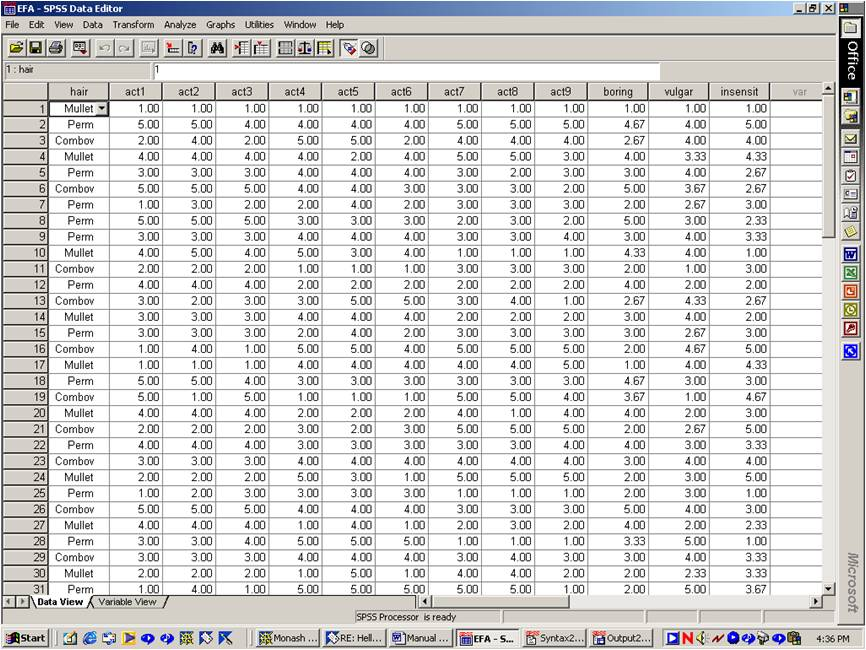### Refinements

Sometimes, the output is less compelling and clear. For example, some of the items may pertain to several factors. In addition, some of the factors may be difficult to interpret. That is, the items that pertain to a particular cluster of variables may not correspond to a common theme. Hence, the substantive meaning of these factors may be unclear. In other words, the factor analysis might need to be amended and then repeated. Indeed, another property of the data might also suggest the factor analyses will need to be repeated. Specifically:

1. The communality of some items might be inadequate.
2. To illustrate the concept of communality, suppose you undertook a regression in which one of the items was the dependent variable and the factors were the independent variables.
3. The communality of an item is essentially the R squared that emerges from this analysis.
4. In other words, communality reflects the extent to which the factors explain the variance of each item.
5. If communality in the extraction column is below 0.4 or so, the items are not explained adequately by the factors. That is, such items do not correspond sufficiently to any of the factors and could thus be discarded.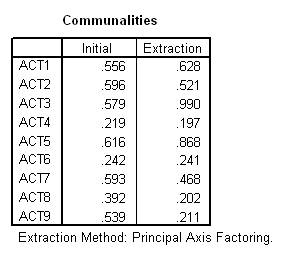The communalities that appear in the column labelled "Initial" are less, if at all, informative. Specifically:

1. To illustrate the rationale that underpins these values, suppose you undertook a regression in which one of the items was the dependent variable and the remaining items were the independent variables.
2. The initial communality of an item is essentially the R squared that emerges from this analysis.
3. In other words, the initial communality reflects the extent to which the variance in each item is explained by the other variables.

The bottom line is that factor analyses often need to be repeated to enhance the solution. In particular, the extraction method, rotation method, the inclusion of variables, and the number of factors can be manipulated. The rationale behind each issue is thus addressed below.

### Extraction method

To understand extraction methods, the theory that underpins factor analysis needs to be appreciated. In particular, factor analysis assumes that individuals vary on a few fundamental traits or attributes, such as the extent to which they are boring, vulgar, insensitive, and so forth. These traits or attributes are called factors. Some hypothetical scores on these traits, together with an extract of the actual data, is provided below.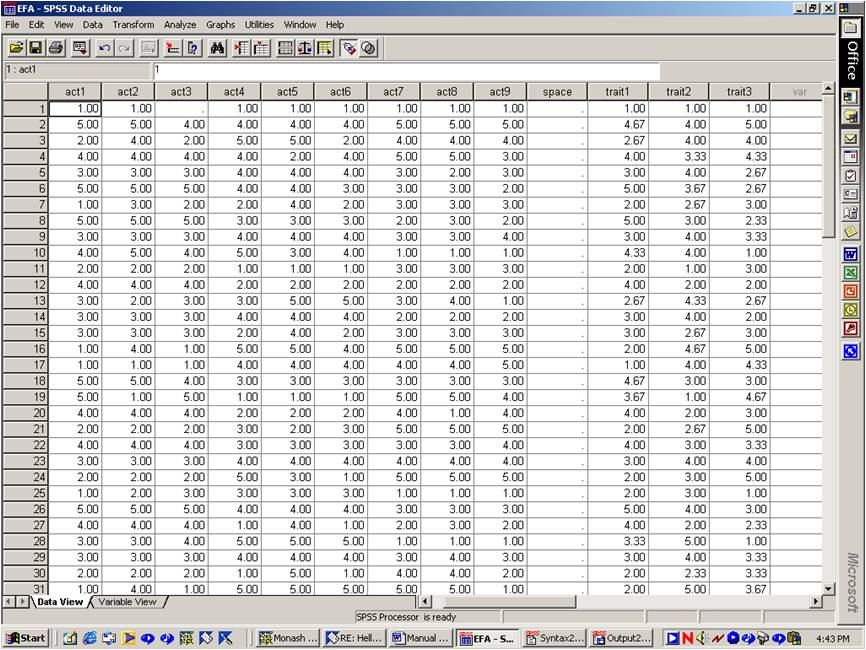Factor analysis assumes the responses on each item are a function of values on the various traits or factors. That is, the relationship between responses on each item and scores on each factor can be represented by a series of equations. Hypothetical equations are presented below. For example, suppose an individual corresponds to a score of 3.8, 1.6, 3.7, and 4.8 for traits 1, 2, 3, and 4 respectively. These scores can be substituted in the following equations to predict their response to each item.

Item 1 = 0.9 x trait 1 + 0.8 x trait 2 + 0.3 x trait 3 + ... + error 1

Item 2 = 0.6 x trait 1 + 0.4 x trait 2 + 0.5 x trait 3 + ... + error 2

Item 3 = 0.1 x trait 1 + 0.1 x trait 2 + 0.1 x trait 3 + ... + error 3

...

Item 9 = 0.4 x trait 1 + 0.5 x trait 2 + 0.7 x trait 3 + ... + error 9

Factor analysis is a process that estimates the coefficients--the numbers that precede each trait or factor. Several techniques, called extraction methods, have been developed to determine these coefficients. For example, alpha factoring is intended to maximise the Cronbach's alpha associated with each factor.

The extraction method called "principal components analysis" assumes these errors equal zero. In other words, responses on each item are entirely dependent upon scores on each trait. The other extraction methods, such as "principal axis factoring", "maximum likelihood", and "alpha factoring" do not assume these errors equal zero--an assumption that is often unreasonable. Indeed, some researchers restrict the term "factor analysis" to the extraction methods that do not assume the errors are zero, and thus exclude principal components analysis from this definition. Indeed, factors are called components whenever principal components analysis is used. In reality, however, all the methods yield comparable results.

### Rationale that underlies the extraction methods

This section outlines the rationale that underpins principal components analysis, principal axis factoring, and some of the other techniques. This rationale does not need to be understood and can be skimmed. To illustrate principal components analysis, consider the correlation matrix again.

Principal components analysis first creates a new column, called component 1. To illustrate, this column is presented in the following data file.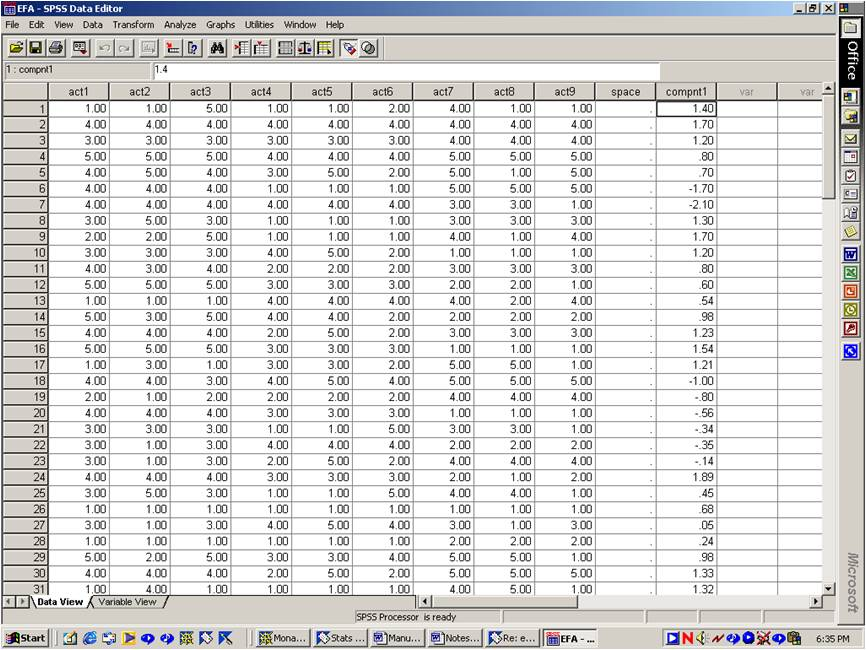This column of numbers is intended to capture the variance in each item. To illustrate this concept, principal component computes a set of equations, such as:

Item 1 = 0.9 x component 1

Item 2 = 0.6 x component 1

Item 3 = 0.1 x component 1

...

Item 9 = 0.4 x component 1

In other words, principal components analysis estimates the scores on each item, using these equations. These estimated scores are illustrated in the following data file.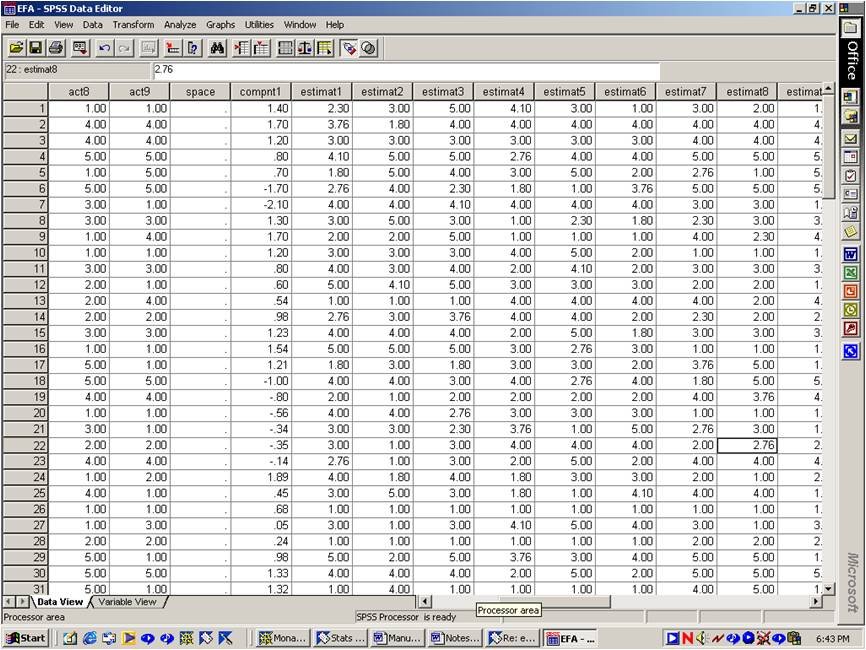The correlation between these items is presented in the following table.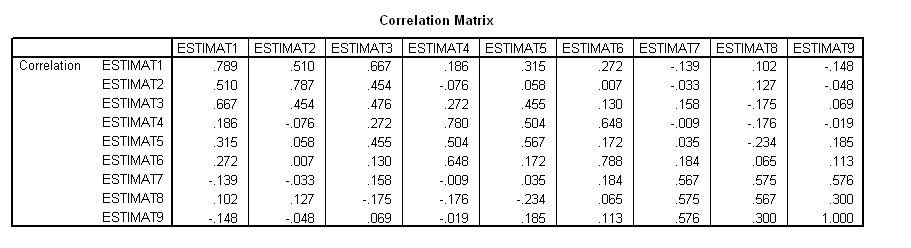This estimated correlation matrix deviates somewhat from the observed correlation matrix, which was presented earlier. In other words,

1. These estimated items are not entirely accurate.
2. That is, these estimated items do not mirror the original items.
3. Hence, principal components analysis continues to add components until this estimated correlation matrix matches the observed, or actual, correlation matrix.
4. The number of components will always equal the number of items.

Principal axis factoring resembles this technique. However, principal axis factoring attempts to match the estimated correlation matrix with a variant of the observed correlation matrix. Specifically, the 1s in the diagonal are replaced with the initial communalities. This adjusted matrix is presented below. This adjustment incorporates some error into the equations, which is more representative of reality.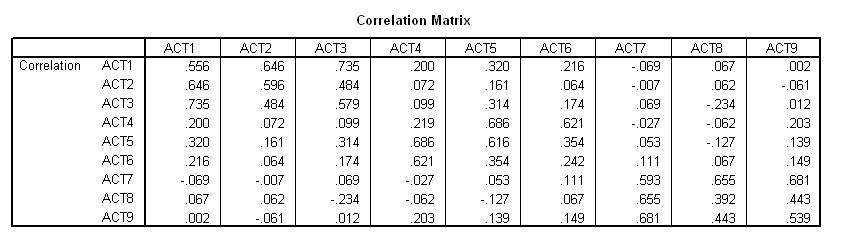The final method that will be discussed is unweighted least squares. This method is seldom utilised, but provides an interesting insight into factor analysis. Specifically, this method attempts to minimise the residuals. Residuals represent the difference between the estimated and actual correlation matrix. The estimated matrix, sometimes called the reproduced matrix, together with the residuals are presented below.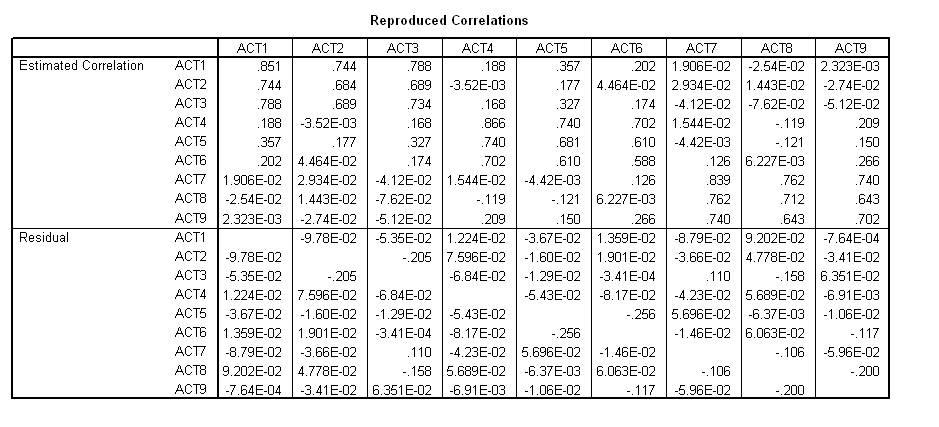Many researchers examine the residual matrix to determine whether or not more factors should be included. In particular:

1. To generate this matrix, undertake factor analysis as usual, but do not press OK
2. Press the "Statistics" button and tick "Reproduced Matrix"
3. Press Continue and then OK

If one or two of the residuals exceed 0.1, or several residuals exceed 0.05, another factor should perhaps be included.

### Rotation method

Many rotation methods have been developed to simplify interpretations. These methods can be subdivided into two sets: orthogonal and oblique. Orthogonal methods, such as varimax, equamax, and quartamax, all yield factors that are uncorrelated. Oblique methods, such as direct oblimin and promax, yield factors that can be correlated.

To illustrate, suppose you could actually measure the traits or factors of each individual. These hypothetical scores are presented below. An orthogonal method would ensure these three columns of factor scores are uncorrelated. That is, high scores on one factor would not necessarily coincide with high scores on another factor. In contrast, an oblique method would not impose this restriction.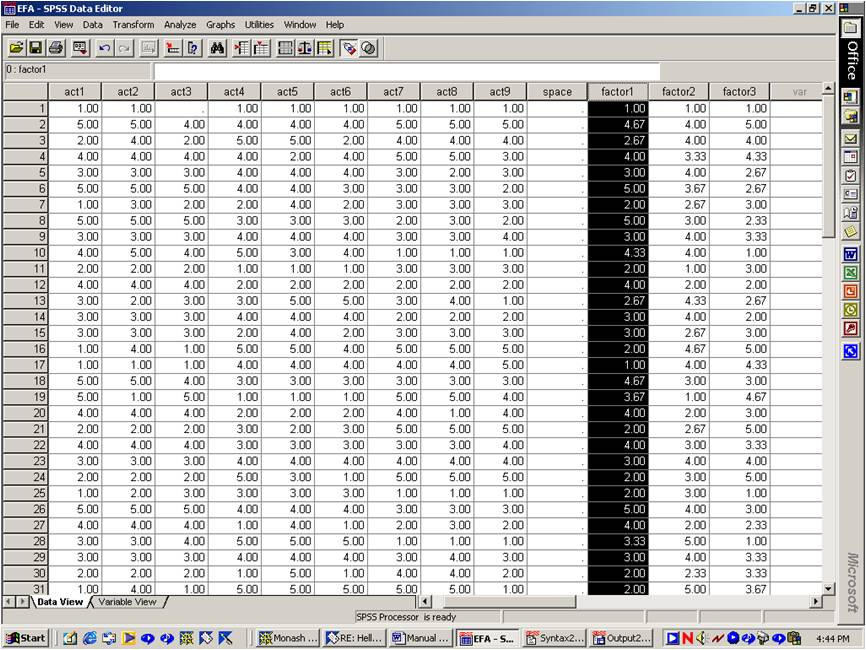To undertake an oblique rotation with SPSS, many researchers specify the method called "Direct oblimin". The researcher must also stipulate a parameter called delta, which represents the extent to which the factors can correlate. A delta of 0 is often chosen.

Oblique methods yield two rather than one rotated matrices, called a structure and pattern matrix respectively. A structure matrix provides the correlation between each item and factor. For example, the table below suggests that Act 1 and Factor 1 yield a correlation of -0.048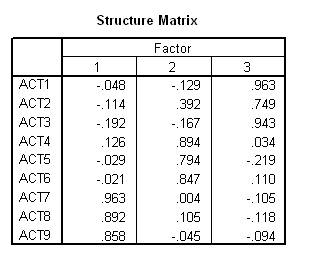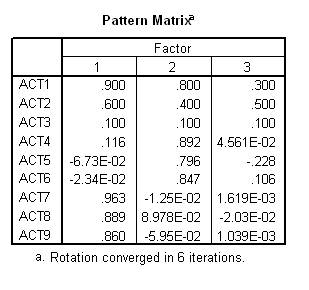The pattern matrix specifies the coefficients that link each item to the various traits or factors. For example, suppose the following equations relate the responses on each item to the factors. In this instance, the coefficient that links item 1 to factor 1 is 0.90, and hence this value would appear in the pattern matrix. Researchers tend to utilise the structure matrix in lieu of the pattern matrix, but not always. When the rotation is orthogonal, the structure and pattern matrix are identical, and thus merely called a rotated matrix.

Item 1 = 0.90 x trait 1 + 0.80 x trait 2 + 0.30 x trait 3 + error 1

Item 2 = 0.60 x trait 1 + 0.40 x trait 2 + 0.50 x trait 3 + error 2

Item 3 = 0.10 x trait 1 + 0.10 x trait 2 + 0.10 x trait 3 + error 3

### Rationale that underpins rotation

This section specifies the rationale that underpins the rotation process and can perhaps be skimmed. To appreciate rotation, consider the following factor matrix.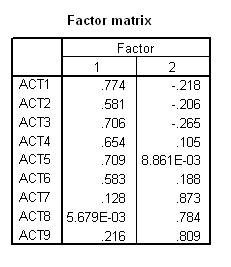This factor matrix can be represented by the following graph. Each point represents a separate factor. For instance, Act 1 pertains to a score of 0.744 on Factor 1 and -0.218 on Factor 2.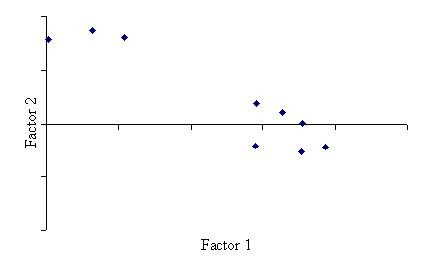The axes can then be rotated, as illustrated below. The new values are represented by the rotated matrix.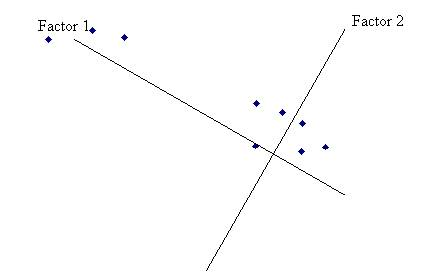Orthogonal methods ensure these axes remain perpendicular to one another. Specifically:

• Varimax rotation maximises the variability of loadings associated with each factor. In other words, the values in each column of the rotated matrix differ substantially from one another.
• Quartimax rotation maximises the variability of loadings associated with each item. In other words, the values in each row of the rotated matrix differ substantially from one another.
• Equamax is a compromise between these two methods.

Oblique methods, such as direct oblimin, do not ensure the axes remain perpendicular to one another. In particular:

• Higher delta values permit smaller angles between the factors
• Indeed, when delta equals 1, the axes might virtually touch
• On the other hand, when delta equals -4, the axes must be virtually perpendicular.

### Item removal

Suppose the researcher has utilised several extraction and rotation methods, but the outcomes remain unclear and unconvincing. They could thus consider removing items that pertain to several factors, complicate the interpretations, or generate a low communality. In other words, the process is supposed to be iterative and subjective.

Ultimately, researchers seek a pattern of results called simple structure. That is, they want each item to correspond to exactly one factor. In addition, they want each factor to correspond to three or more items.

### Illustration of the format used to report factor analysis.

The nine acts were subjected to principal axis factoring with a varimax rotation. Table 1 presents the rotation matrix that emerged, together with the eigenvalue, percentage of variance after rotation, and Cronbach's alpha associated with the three primary factors.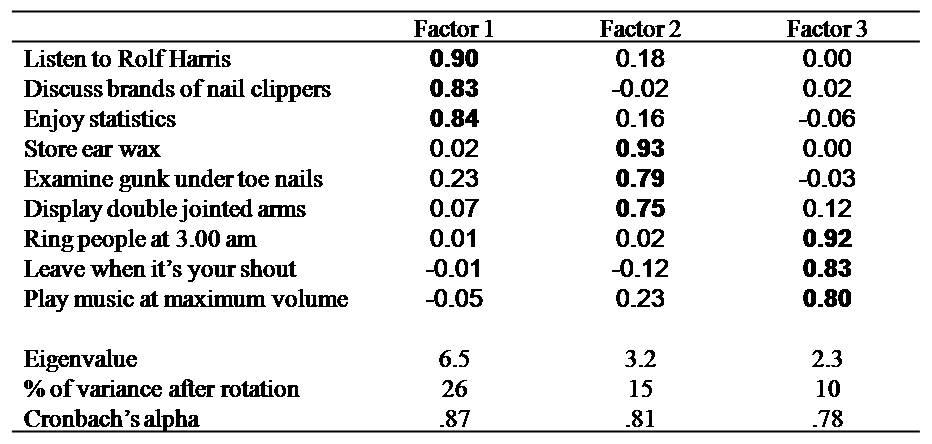The first factor comprises items that perhaps reflect the extent to which the individuals are dull, and thus will be labelled "boring". The second factor entails items that represent the degree to which the individuals are vulgar and will therefore be referred to as "gross". Finally, the third factor includes items that reflect insensitive behaviour, and will thus be labelled "selfish".